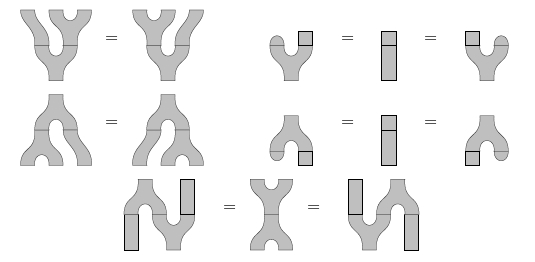# Gauss Sums over "semisimple spherical tensor category"?

I read on the arXiv the following:

Let $\mathcal{\mathbf{C}}$ be a semisimple spherical tensor category with simple unit and let $\mathbf{\Gamma}$ be the set of isomorphism classes of simple objects.

Unfortunately I could not read further since I didn't understand the jargon:

• category: a collection of objects with morphisms satisfying certain rules.
• e.g. the category Set of Sets and functions between them.
• tensor category has a notion of "tensor product" $\otimes$
• e.g. Vect the category of vector spaces and linear maps between them
• spherical tensor category
• A spherical category is a monoidal category with duals that behaves as if its morphisms can be drawn and moved around on a sphere. Confused
• A spherical category is a pivotal category where the left and right trace operations coincide on all objects. Even more so since I don't know what "pivot" means or why there is left and right "trace"
• pivotal category
• A pivotal category is an autonomous category equipped with a monoidal natural isomorphism A→(A∗)∗. Pivotal categories have also been called “sovereign categories.” This is a kind of category with duals.
• E.g. (possibly?) Vect with the duality operation $(V^\ast)^\ast = V$
• semicimple category
• A semisimple category is a category in which each object is a direct sum of finitely many simple objects, and all such direct sums exist.
• E.g. Category of representation of a finite group $G$ which have notions of tensor product $\otimes$ and direct sum $\oplus$, so they behave almost like a ring.

Another look through the paper suggests I am looking for a Frobenius algebra, which has zany rules like these:Now I can read the second half the sentence:

If $\mathbf{\Gamma}$ is finite we can define $\dim \mathbf{\mathcal{C}} = \sum_{i \in \Gamma} d(X_i)^2$

Because the category is irreducible every object has a reduction to the direct sum of simple object. Presumably there is a way to compute these dimensions of these things? Continuing...

If $\mathcal{\mathcal{C}}$ is finite dimensional and braided then the Gauss sums of $\mathcal{\mathbf{C}}$ are defined by $$\Delta_{\pm} \mathcal{\mathcal{C}} = \sum_{i \in \Gamma} \omega(X_i)^{\pm 1} d(X_i)^2$$ where $\theta(X) = \omega(X) id_X$ is the twist of the simple object $X$ defined by the spherical structure.

So I look up braided monoidal category the tensor product $\otimes$ has to satisfy "hexagon rules"...

What is an example of a finite dimensional braided spherical tensor cateogry ? And how do I compute each term in the sum above in such an instance?

• I don't even understand how this corresponds to the classical Gauss sum, if $\mathcal{C}$ is the category of representations of $\mathbb{Z}/p\mathbb{Z}$ or the appropriate group May 30, 2014 at 14:14

Definitions.

1. Let $A$ be a finite abelian group. A quadratic form on $A$ is a map $q: A \to \mathbb{C}^{\times}$ such that $q(-a) = q(a)$, and $\langle a, b \rangle = \frac{q(a + b)}{q(a)q(b)}$ is a biadditive form. We call a pair $(A, q)$ a pre-metric group.
2. A fusion category is pointed if every simple object is invertible. (This implies that the dimension of every simple object is 1. Such a category is necessarily spherical in a trivial way.)

The following theorem is contained (with slightly modified wording) in Joyal and Street, 1986, though the result essentially dates back to Eilenberg and Mac Lane in 1950 (albeit without the language of tensor categories).

Theorem. Given a pre-metric group $(A, q)$, there is a unique braided pointed fusion category $\mathcal{C}(A, q)$ such that

1. simple objects are indexed by $A$;
2. tensor product comes from the abelian group structure of $A$; and
3. the braiding on $a \otimes a$ satisfies $c_{a, a}^2 = q(a)$.

Furthermore, every braided pointed fusion category is equivalent to one of this form.

From 3, it follows, that $$\Delta_\pm \mathcal{C}(A, q) = \sum_{a \in A} q(a)^{\pm 1}.$$ To recover the classical quadratic Gauss sums, take $A = \mathbb{Z} / p\mathbb{Z}$ and $q(a) = \zeta^{a^2}$ for $\zeta$ a $p$th root of unity.

A more thorough treatment of Gauss sums for the categories coming from pre-metric groups can be found in Chapter 6 and Appendix A of On braided fusion categories I by Drinfeld, Gelaki, Nikshych, and Ostrik.

• When are dimensions of simple objects not $1$ or not integers? May 31, 2014 at 11:24
• I was missing that these are multiplicative characters $q(x,y) = e^{2\pi i (x^2 + y^2)}$ or such. Jun 7, 2014 at 14:17